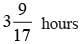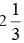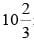# Olympiad Test: Elementary Mensuration-II - 2

## 20 Questions MCQ Test Mathematics Olympiad Class 7 | Olympiad Test: Elementary Mensuration-II - 2

Description
Attempt Olympiad Test: Elementary Mensuration-II - 2 | 20 questions in 40 minutes | Mock test for Class 7 preparation | Free important questions MCQ to study Mathematics Olympiad Class 7 for Class 7 Exam | Download free PDF with solutions
QUESTION: 1

### What is the capacity of the cylindrical tank? I. The area of the base is 61,600 sq. cm. II. The height of the tank is 1.5 times the radius. III. The circumference of base is 880 cm.

Solution:

Capacity = πr2h
From I, πr2 = 61600. This gives r.
From II, h = 1.5 r Thus, I and II give the answer.
Again, III gives 2 πr = 880. This gives r.
So, II and III also give the answer.

QUESTION: 2

### Three pipes A, B and C can fill an empty tank fully in 30 minutes, 20 minutes, and 10 minutes respectively. When the tank is empty, all the three pipes are opened. A, B and C discharge chemical solutions P, Q and R respectively. What is the proportion of the solution R in the liquid in the tank after 3 minutes?

Solution:

Part filled by (A + B + C) in 3 minutes = 3 (1/30 + 1/20 + 1/10) = (3 × 11/60) = 11/20
Part filled by C in 3 minutes = 3/10
∴ Required ratio = (3/10 × 20/11) = 6/11

QUESTION: 3

### Pipes A and B can fill a tank in 5 and 6 hours respectively. Pipe C can empty it in 12 hours. If all the three pipes are opened together, then the tank will be filled in

Solution:

Net part filled in 1 hour (1/5 + 1/6 – 1/12)
= 17/60
∴ The tank will be full in 60/17 hours i.e.,QUESTION: 4

A pump can fill a tank with water in 2 hours. Because of a leak, it tookhours to fill the tank. The leak can drain all the water of the tank in:

Solution:

Work done by the leak in 1 hour
= (1/2 – 3/7) =1/14
∴ Leak will empty the tank in 14 hrs.

QUESTION: 5

Two pipes A and B can fill a cistern in 37.5 minutes and 45 minutes respectively. Both pipes are opened. The cistern will be filled in just half an hour, if B is turned off after:

Solution:

Let B be turned off after x minutes. Then,
Part filled by (A + B) in x min. + Part filled by
A in (30 – x) min. = 1.
∴ x( 2/75 + 1/45) + (30 – x)2/75 = 1
⇒ 11x/225 + (60 – 2x)/75 = 1
⇒ 11x + 180 – 6x = 225
⇒ x = 9

QUESTION: 6

A tank is filled by three pipes with uniform flow. The first two pipes operating simultaneously fill the tank in the same time during which the tank is filled by the third pipe alone. The second pipe fills the tank 5 hours faster than the first pipe and 4 hours slower than the third pipe. The time required by the first pipe is:

Solution:

Suppose, first pipe alone takes x hours to fill the tank .
Then, second and third pipes will take (x – 5) and (x – 9) hours respectively to fill the tank.
1/x + 1/(x – 5) = 1/(x – 9)
(x – 5 + x)/x(x – 5) =1/(x – 9)
⇒ (2x – 5)(x – 9) = x(x – 5)
⇒ x2 – 18x + 45 = 0
⇒ (x – 15)(x – 3) = 0
⇒ x = 15 [Neglecting x = 3]

QUESTION: 7

Two pipes can fill a tank in 20 and 24 minutes respectively and a waste pipe can empty 3 gallons per minute. All the three pipes working together can fill the tank in 15 minutes. The capacity of the tank is:

Solution:

Work done by the waste pipe in 1 minute
=1/15 – (1/20 + 1/24)
= (1/15 – 11/20)
= – 1/40 [–ve sign means emptying]
∴ Volume of 1/40 part = 3 gallons
Volume of whole = (3 × 40) gallons
= 120 gallons

QUESTION: 8

A tank is filled in 5 hours by three pipes A, B and C. The pipe C is twice as fast as B and B is twice as fast as A. How much time will pipe A alone take to fill the tank?

Solution:

Suppose pipe A alone takes x hours to fill the tank.
Then, pipes B and C will take x/2 and x/4 hours respectively to fill the tank.
1/x + 2/x + 4/x = 1/5
7/x = 1/5
⇒ x = 35 hrs

QUESTION: 9

Two pipes A and B together can fill a cistern in 4 hours. Had they been opened separately, then B would have taken 6 hours more than A to fill the cistern. How much time will be taken by A to fill the cistern separately?

Solution:

Let the cistern be filled by pipe A alone in x hours.
Then, pipe B will fill it in (x + 6) hours.
1/x + 1(x + 6) = 1/4
(x + 6 + x)/x (x + 6) = 1/4
⇒ x2 – 2x – 24 = 0
⇒ (x – 6)(x + 4) = 0
⇒ x = 6
[Neglecting the negative value of x]

QUESTION: 10

Two pipes A and B can fill a tank in 20 and 30 minutes respectively. If both the pipes are used together, then how long will it take to fill the tank?

Solution:

Part filled by A in 1 min =1/20
Part filled by B in 1 min =1/30
Part filled by (A + B) in 1 min
= (1/20 + 1/30) =1/12
∴ Both pipes can fill the tank in 12 minutes.

QUESTION: 11

Two pipes A and B can fill a tank in 15 minutes and 20 minutes respectively. Both the pipes are opened together but after 4 minutes, pipe A is turned off. What is the total time required to fill the tank?

Solution:

Part filled in 4 minutes = 4(1/15 + 1/20)
= 7/15
Remaining part = (1 – 7/15) = 8/15
Part filled by B in 1 minute = 1/20
∴ 1/20 : 8/15 : : 1: x
x = (8/15 × 1 × 20) =min
= 10 min. 40 sec.
The tank will be full in (4 min. + 10 min. + 40 sec.) = 14 min. 40 sec.

QUESTION: 12

One pipe can fill a tank three times as fast as another pipe. If together the two pipes can fill the tank in 36 minutes, then the slower pipe alone will be able to fill the tank in:

Solution:

Let the slower pipe alone fill the tank in x minutes.
Then, faster pipe will fill it in x/3 minutes.
1/x + 3/x = 1/36
⇒ 4/x = 1/36
⇒ x = 144 min.

QUESTION: 13

A large tanker can be filled by two pipes A and B in 60 minutes and 40 minutes respectively. How many minutes will it take to fill the tanker from empty state if B is used for half the time and A and B fill it together for the other half?

Solution:

Part filled by (A + B) in 1 minute
= ( 1/60 + 1/40) =1/24
Suppose the tank is filled in x minutes.
Then, x/2( 1/24 + 1/40) = 1
x/2 × x/15 =1
x = 30 min.

QUESTION: 14

A tap can fill a tank in 6 hours. After half the tank is filled, three more similar taps are opened. What is the total time taken to fill the tank completely?

Solution:

Time taken by one tap to fill half of the tank = 3 hrs.
Part filled by the four taps in 1 hour
= (4 × 1/6) = 2/3
Remaining part = (1 – 1/2) = 1/2
∴ 2/3 : ½ :: 1 : x
⇒ x = (1/2 × 1 × 3/2) = 3/4 hours = 45 minutes
So, total time taken = 3 hrs. 45 minutes

QUESTION: 15

Three taps A, B and C can fill a tank in 12, 15 and 20 hours respectively. If A is open all the time and B and C are open for one hour each alternately, the tank will be full in:

Solution:

(A + B)’s 1 hour’s work = (1/12 + 1/15)
= 3/20
(A + C)’s hour’s work = (1/12 + 1/20)
= 2/15
Part filled in 2 hrs = (3/20 + 2/15) = 17/60
Part filled in 6 hrs = (3 × 17/60) = 17/20
Remaining part = (1 – 17/20) = 3/20
Now, it is the turn of A and B and 3/20 part is filled by A and B in 1 hour.
∴ Total time taken to fill the tank
= (6 + 1) hrs = 7 hrs.

QUESTION: 16

Three pipes A, B and C can fill a tank in 6 hours. After working at it together for 2 hours, C is closed and A and B can fill the remaining part in 7 hours. The number of hours taken by C alone to fill the tank is:

Solution:

Part filled in 2 hours = 2/6 = 1/3
Remaining part = (1 – 1/3) = 2/3
∴ (A + B)’s 7 hour’s work = 2/3
(A + B)’s 1 hour’s work = 2/21
∴ C’s 1 hour’s work = {(A + B + C)’s 1 hour’s work} – {(A + B)’s 1 hour’s work}
= (1/6 – 2/21) = 1/14
∴ C alone can fill the tank in 14 hours.

QUESTION: 17

How much time will the leak take to empty the full cistern?
I. The cistern is normally filled in 9 hours.
II. It takes one hour more than the usual time to fill the cistern because of a leak in the bottom.

Solution:

I. Time taken to fill the cistern without leak = 9 hours.
Part of cistern filled without leak in 1 hour = 1/9
II. Time taken to fill the cistern in presence of leak = 10 hours.
Net filling in 1 hour =1/10
Work done by leak in 1 hour = (1/9 – 1/10) =1/90
∴ Leak will empty the full cistern in 90 hours.
Clearly, both I and II are necessary to answer the question.

QUESTION: 18

How long will it take to empty the tank if both the inlet pipe A and the outlet pipe B are opened simultaneously?
I. A can fill the tank in 16 minutes.
II. B can empty the full tank in 8 minutes.

Solution:

I. A’s 1 minute’s filling work = 1/16
II. B’s 1 minute’s filling work =1/8
(A + B)’s 1 minute’s emptying work
= (1/8 – 1/16) =1/16
∴ Tank will be emptied in 16 minutes.
Thus, both I and II are necessary to answer the question.

QUESTION: 19

If both the pipes are opened, how many hours will be taken to fill the tank?
​I. The capacity of the tank is 400 litres.
II. The pipe A fills the tank in 4 hours.
III. The pipe B fills the tank in 6 hours.

Solution:

II. Part of the tank filled by A in 1 hour = 1/4
III. Part of the tank filled by B in 1 hour = 1/6
(A + B)’s 1 hour’s work = (1/4 + 1/6) = 5/12
∴ A and B will fill the tank in 12/5hrs
= 2 hrs 24 min.
So, II and III are needed.

QUESTION: 20

Find the number of lead balls of diameter 1 cm each that can be made from a sphere of diameter 16 m.

Solution:

Number of balls = Volume of big sphere /
Volume of one small sphere
= (4 π / 3 × 8 × 8 × 8)/
(4 π / 3 × 0.5 × 0.5 × 0.5)
= 4096Use Code STAYHOME200 and get INR 200 additional OFF Use Coupon Code